## Main

The charge of the antihydrogen atom can be inferred from the charge anomalies of the antiproton and positron. The charge anomaly of the antiproton is known from spectroscopic measurements8,10 on antiprotonic helium,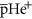, and is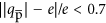parts per billion (p.p.b.) with a confidence level of 90%, where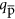is the antiproton charge. The charge anomaly of the positron8,9,11 is less well known: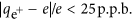, where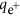is the positron charge (no confidence level given, but assumed here to be 1σ), determined by measurements of the positron cyclotron frequency and the positronium Rydberg constant. Assuming charge superposition, we can therefore combine these two anomalies to infer an indirect experimental bound of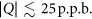on the charge Qe of antihydrogen. This bound is much looser than the direct experimental bounds for normal-matter atoms; however, the methods used to set these normal-matter bounds generally use macroscopic quantities of atoms and so are unsuitable for studies of antihydrogen, of which only approximately 1,000 antiatoms have been trapped and detected1,2,3,4.

The earliest direct experimental limit on the charge of antihydrogen, |Q| < 0.02, came from the observation12 that Lorentz forces were insufficient to deflect an energetic (momentum of 1.94 GeV c−1) beam13 of antihydrogen atoms away from a detector. A much more precise bound, Q = (−13 ± 11 ± 4) p.p.b. (1σ confidence level), was recently determined5 by searching for a static-electric-field-induced shift in the average position of antiatoms confined in the ALPHA-I antihydrogen trap at CERN. This shift was measured by recording the position along the electric-field axis as the antiatoms were released from the trap by turning off the trapping magnetic fields.

The precision of this static shift measurement was limited by the magnitude of the applied electric fields, and by statistical and systematic issues related to accurately measuring small deflections. The sensitivity of the charge measurement can be enhanced by using stochastic (that is, randomly time-varying) electric fields5,14,15. Under the influence of such fields, a putatively charged, trapped antihydrogen atom would experience small, stochastic energy kicks. On average, the antiatom would gradually gain enough energy from these kicks to escape the shallow potential well in which it was trapped. This process can be modelled as a random walk similar to Fermi acceleration16. By searching for the loss of antiatoms caused by these stochastic electric fields, we set a new limit on |Q|.

Our measurement was performed on antihydrogen atoms confined in the ALPHA-2 trap at CERN. This trap uses a minimum-magnetic-field (minimum-B) configuration to trap the antiatoms. Schematics of the trap and fields are shown in Fig. 1a and b, respectively. The magnetic minimum is formed by mirror and octupole magnetic coils. Antihydrogen atoms whose magnetic moments are oriented antiparallel to the trap magnetic fields are attracted to the magnetic minimum at the trap centre; for our fields, which are of the order of 1 T, the depth of the antihydrogen potential well is Ewell = 0.54 K, where Ewell is measured in temperature units.

The general methods by which we trap antihydrogen are described elsewhere1,2,3,17, but briefly, antihydrogen atoms are created by first trapping separate cold plasmas containing several million positrons and several tens of thousands of antiprotons. The positrons come from a radioactive sodium source18, and the antiprotons are generated by CERN’s Antiproton Decelerator (AD). Antiatoms are formed by three-body recombination when these two plasmas are mixed using autoresonance19,20 in the trap interior. Approximately 0.01% of the roughly ten thousand atoms created in each 1-s-long mixing interval are sufficiently cold to be trapped. The antiatoms are detected by releasing them from the trap by turning off the trap magnetic fields. A three-layer silicon vertex detector (SVD) surrounding the trap is used to detect, locate and time the resulting antiatom annihilations21. The detector and its associated multivariate analysis22,23,24 (MVA) software can detect approximately 82% of the antiatoms that annihilate in the trap.

To generate the stochastic electric field necessary for our measurement, we impose the electric potential shown in Fig. 1c on the trap by biasing the trap electrodes. We make this potential, and the associated electric field, vary stochastically by repeatedly changing the bias on the electrodes from that shown in Fig. 1c to its negation. We use N = 84,900 transitions, which, altogether, last 114.9 s in each experimental cycle (see Fig. 2). The interval between transitions averages 1 ms, but is randomized for reasons that are discussed later.

Each of the potential transitions would non-adiabatically perturb the kinetic energy of a charged antiatom; as noted above, these kicks correspond, approximately, to a random walk in energy. To model this process, we need to estimate the average potential change subsequent to each of the transitions. Measurements using our first trap, ALPHA-I3, showed that the energy of the trapped antiatoms is roughly distributed2,5,25,26 as a high-temperature, three-dimensional (3D) Maxwellian distribution truncated near Ewell. The typical energy of the component of motion along the trap axis is therefore about 0.1 K, which corresponds to a travel distance of about 30 mm in 1 ms. From the purple brackets shown in Fig. 1c, we see that an antiatom possessing this axial kinetic energy would experience a potential change of ΔΦ ≈ 100 V in 1 ms, although there are substantial uncertainties in this estimate due to variations in the potential over the volume of the trap, the distribution of kinetic energies and the potential changes arising from radial motion. A simple energy-diffusion model predicts that an antiatom with a putative charge Qe would gain energy of about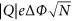. Consequently, the antiatom would gain roughly enough energy to escape from a trap of depth Ewell if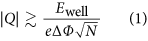Evaluating this equation using our experimental parameters yields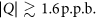However, equation (1) assumes that the antiatom starts with zero initial kinetic energy. Prior measurements indicate that the average antiatom starts with more than half the energy it needs to escape; thus, the bound on |Q| is probably lower.

Stochastic acceleration requires an element of randomness in the relation between the frequency of the driving-potential inversions and the frequencies of the antiatom orbital oscillations. Trajectory simulations (see, for example, figure 6 in ref. 15) demonstrate that the orbits in the unforced system are generally not regular and do not have a fixed frequency. In many cases, these variations in orbital frequency would make even a strictly periodic driving potential act stochastically. However, some orbits have long-lasting periods of regular motion, and antiatoms in these orbits might not be accelerated by the driving potential. As proposed in ref. 15, we introduce sufficient stochasticity into the system by a random modulation, with a uniform distribution and a standard deviation of 0.2 ms, of the time interval between potential transitions. (This variability is visible in Fig. 2b–d.) Simulations14,15 show that it is not necessary or beneficial to randomize the voltage levels between which the electrode potentials switch.

To bound |Q|, we alternated between two experimental protocols, each with four phases. The first protocol involved stochastic trials, in which we (1) trapped antihydrogen, (2) subjected any trapped antihydrogen to the stochastic potential variations described above, (3) allowed any antiatoms remaining after the stochastic phase to escape by turning the confining magnetic fields off, and then (4) counted the number of antiatoms that thus escaped using the silicon vertex detector. The second protocol involved null trials, which were performed in strict alternation with the stochastic trials, and were identical to them in all respects, except that the potentials were set to zero during phase (2). Thus, in both types of trials we attempted to hold the antiatoms for the same time (114.9 s) after phase (1). Assuming that the underlying initial antihydrogen trapping rate and all other systematic effects are the same in the stochastic and null trials, a statistically significant difference between the number of antiatoms detected after these trials would imply the existence of a non-zero Q being acted upon by the oscillating electric fields of the stochastic trials. (As discussed in Methods, known polarization and polarizability effects are negligible in the current experiment.)

The results of executing both protocols ten times are presented in Table 1. We observed no differences in the number of antiatoms released in phase (3) between the two protocols, which suggests that the charge—if any—of antihydrogen is below that required to escape from the trap,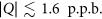(based on the analytic estimate given above). This rough estimate can be made more robust and precise by simulating the dynamics of the antiatoms and using a proper statistical analysis. (Events detected during phase (2) are compiled in Extended Data Table 1 and discussed in Methods, and are compatible with cosmic rays.)

We use simulations1,2,5,15,25,26 that model the experiment closely, including detailed models of the trap fields and stochastic timing, and realistic initial conditions validated by previous experiments. The simulations begin by mimicking the initial mixing of the two plasmas and subsequent relaxation periods. We model the null trials by propagating the antiatoms in the electric-field-free trap for a further 114.9 s. We model the stochastic trials by assigning the antiatoms a putative Q, and propagating them in the presence of the stochastic electric fields for this same 114.9 s. For each value of Q studied, we launch 1,000 antiatoms.

For each simulated Q, we determine the survival probability s by calculating the ratio of the number of antiatoms that survive the stochastic simulations to the number that survive the null simulations; the results are shown in Fig. 3. Because the stochastic drive reverses direction every half-cycle, it is unbiased on average; consequently, s depends on the magnitude of the charge |Q|, but not on its sign.

Although it is clear from Fig. 3 that the bound on |Q| set by our experiment will be in the neighbourhood of 1 p.p.b., it is not immediately clear exactly what s and, hence, what |Q| to assign from this figure and the data in Table 1. We might begin by setting a threshold of s = 0.5; however with a per-trial antiatom observation rate of r ≈ 24/20 = 1.2, the probability that 12 (what we observed) or more antiatoms would have survived in 10 trials with s = 0.5 is only 0.02 assuming Poissonian statistics. Clearly, our data will support setting the threshold at some larger value of s. Furthermore, our limited data set only provides an estimate for r. This suggests that a Bayesian methodology, in which r is treated as a nuisance parameter, would be useful for finding a more precise limit on |Q|.

In Methods we present a simple Bayesian analysis that suggests that s > 0.79 and that the corresponding bound on |Q|, found by inverting the curve in Fig. 3, is |Q| < 0.59 p.p.b. However, this analysis ignores systematic uncertainties; fortunately, our experiment is relatively insensitive to these uncertainties. For example, the analytic expression given in equation (1) shows that uncertainties in Φ (or in the magnitude of the electric field |E|) affect our bound on |Q| linearly. Because these fields are known to within approximately 1%, uncertainties in Φ have a negligible effect compared to that of our statistical uncertainties. Similarly, uncertainties in the magnitude of the magnetic field |B| contribute to uncertainties in |Q| through its linear dependency on Ewell. Because Ewell depends on the mirror and octupole fields approximately linearly, and because both are known to within approximately 1%, these effects are unimportant. Details of the magnetic field structure beyond the maximum and minimum are similarly unimportant because they do not affect the trap depth. Simulations show that s is not greatly influenced by changes in the volume from which the antiatoms are initially launched.

All of the data were taken over adjacent half-shifts, separated by 16 h, at CERN’s AD facility. We are not aware of any mechanism that could have substantially altered the observation rate r in synchronization with the strict alternation of the stochastic and null trials. Likewise, this alternation makes the measurement robust against failures of the detector system. Nonetheless, we performed extensive tests27 of the detector performance to confirm that there were no substantial failures or performance degradations (see Extended Data Fig. 1). The expected number of cosmic rays incorrectly identified as antiatoms is 0.07 for each of the two sets of ten trials; this has a negligible effect on our bound on |Q| (see Supplementary Information).

Simulations do reveal a small dependence of s on the initial energy distribution of the antiatoms. Two distributions that bound our preferred, truncated Maxwellian distribution were studied for our previous apparatus ALPHA-I. Although we have good evidence to rule out these bounding distributions5,25,26, their impact on the measurement reported here is discussed in Supplementary Information. With an additional, even smaller correction arising from the statistical error in the Monte Carlo simulation, our bound degrades to |Q| < 0.71 p.p.b. at a 68.3% (1σ) confidence level. This bound represents a 20-fold improvement on the best previous bound5.

Given that the charge anomaly of the antiproton8,10 is bound at below 0.7 p.p.b., our result, combined with the assumption of charge superposition, limits the charge anomaly of the positron to 1 p.p.b. (1σ), a 25-fold improvement on the best previous bound8,9. By modestly increasing the potentials, holding the antiatoms for longer times and cooling the antiatoms28, we expect (see the scalings in equation (1)) to be able to improve the antihydrogen charge bound by several orders of magnitude15; these realizable improvements exceed those that could be obtained by the previously used static-electric-field shift methodology5.

## Methods

### Stochastic potentials

The stochastic potentials are applied by biasing the electrically isolated trap electrodes shown in Fig. 1a. Within the approximately 280-mm-long trapping region, we impose roughly 2.25 spatial periods of an electric potential that varies between approximately ±100 V (see Fig. 1c). These potentials come in fifty time-identical repetitions (see Fig. 2a). Individual repetitions last 1.698 s and are spaced at intervals of 2.298 s. Each repetition begins and ends with the potentials zeroed. Within each repetition, the potential cycles between that shown in Fig. 1c and its negation; the average time interval between transitions is 1 ms. Thus, there are 1,698 transitions in each repetition and N = 84,900 transitions in total. Typical time histories of the potentials are shown in Fig. 2b–d. The transitions begin in synchronization for all electrodes; the differences between the attacks (the rise times of each pulse) and magnitudes of the three potentials shown in Fig. 2b–d are due to deliberate differences in the amplifiers and filters driving the electrodes. These differences are precisely modelled in our simulations, and are not otherwise germane to the results presented here.

### Data collection

All data were collected in two adjacent half-shifts, separated by an off-shift period, at CERN’s AD. The data collection followed the protocol outlined above of strict alternation of stochastic and null trials. Data collection ended when we reached the predetermined number (ten) of trials of each type. Trials that had an immediately obvious failure (no antiprotons delivered from the AD, premature failure of our magnet system and so on) were aborted and repeated; otherwise, all the data that were collected are presented here.

### Equation of motion

The antiatom equation of motion in the trap is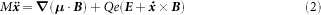Here, x = x(t) is the centre-of-mass position of an antiatom at time t, E = E(x, t) and B = B(x) are the applied electric and magnetic fields, M is the total mass and μ is the magnetic moment of antihydrogen. A trapped antiatom must be low-B-field-seeking, so the direction of μ is assumed to adiabatically track the direction antiparallel to B(x(t)).

### Simulations

The simulations mimic the experimental procedures closely, and are similar to those in refs 1, 2, 5, 14, 15, 25 and 26, in which numerous tests of robustness of the dynamical model and numerical approximations have been performed. Simulated antiatoms are launched from a volume corresponding to the precursor positrons. The antiatoms are drawn from an energy distribution obeying a truncated 3D Maxwellian, which scales as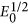at low energies, where E0 is the initial energy of the antiatoms. The truncation energy is set to 0.75 K, well above the trap depth of Ewell = 0.54 K, but well below the positron temperature of tens of kelvin that sets the overall Maxwellian temperature. As discussed below, the antiatoms are then held for 1.2 s. Most of the antiatoms with energies above Ewell will be lost during this relaxation/randomization time; however, a few, on quasi-bound orbits, will remain2,29.

In the experiment, we mix positrons and antiprotons for 1 s. Antihydrogen production peaks at the beginning of the mixing phase, but we do not know when within the mixing phase the antihydrogen that is trapped is created. After mixing, the antiatoms are allowed to randomize for 0.4 s. During this relaxation/randomization phase, electric fields are applied that clear the positrons and antiprotons remaining in the trap. We do not model these fields because they are generally weak compared to the stochastic potentials, there are few transitions and, experimentally, both the stochastic and null trials experience these fields. Altogether, these phases take a total of 1.4 s; in the simulations, to account for the uncertainty in the synthesis time, we allot 1.2 s for this composite relaxation/randomization time. We note that 1.2 s is short compared to the 114.9-s stochastic/hold phase, and that relaxation continues during this phase. Thus, the 0.2-s uncertainty has a negligible effect on our results.

We launch 1,000 antiatoms for each case we study. About 67% of these antiatoms survive the initial relaxation phase. Because 61% have initial energy below Ewell, the remaining 6% are on quasi-bound orbits.

As discussed in the main text, we then propagate the antiatoms remaining after the relaxation phase for 114.9 s without the stochastic electric fields (null trials), or with the stochastic fields and with an assumed Q (stochastic trials). At the end of the 114.9 s, we count the number of antiatoms that have survived the simulation to determine the survival probability; there is no need to model the magnetic field shut-off used to count the antiatoms in the experiment.

We use a symplectic leap-frog propagator to numerically integrate equation (2). The electric potentials in the simulation are determined from a precise model of the trap geometry, using the COMSOL finite element code30 to solve the Laplace equation, and the measured time-history of the potentials on each electrode (see Fig. 2). The magnetic field is calculated from high-accuracy analytic expansions derived from numerical Biot–Savart modelling25. We do not see any interesting effects from coarsening the field solutions (see also ref. 5).

Simulation convergence studies show that the step size need not be shorter than 4 μs; we used 0.8 μs for the simulations reported here. They also indicate that the electrode drive amplifier response time need only be known to the 4-μs level, which is well within its measured accuracy.

### Bayesian analysis

In the Supplementary Information, we present a complete Bayesian analysis that explicitly accounts for uncertainty in the relationship between s and |Q| due to the finite number of samples in our Monte Carlo simulations, and for various systematic effects. Here, we give an abbreviated analysis. We search for the value of the survival probability, s*, that yields a (1 − α) posterior credible interval given our observations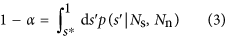Here, p(s | Ns, Nn) is the probability density for the survival probability s, given a total of Ns observed atoms in the stochastic trials and Nn observed atoms in the null trials, and 1 − α = 0.683 for the equivalent of a 1σ credible interval. Bayesian formalism gives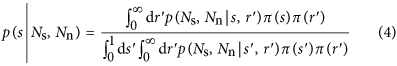where π(s) and π(r) are the priors on s and r, respectively. The likelihood p(Ns, Nn | s, r) is given by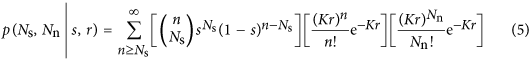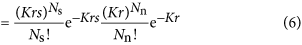where K = 10 is the number of stochastic and null trials. The first factor in the summation in equation (5) is a binomial subsampling of the Poisson-distributed number (given by the second factor) of antiatoms n present in the stochastic trials. (The Poisson assumption is further discussed in the Supplementary Information.) This subsampling models loss, with probability 1 − s, due to the stochastic fields. The third factor is the Poisson-distributed number of antiatoms present in the null trials. The first two terms can be combined into a single Poisson process, yielding equation (6).

The bound on |Q| compatible with the current experiment is substantially lower than the previously reported bound5, and we do not possess accurate prior information about the actual observation rate r in the particular trials used here. Hence, we choose to use uninformative priors. Various notions of uninformativeness have been suggested31. For both binomial and Poisson sampling distributions, the widely used Bernardo–Berger reference priors32, Jeffreys invariant priors33 and Rodriguez entropic priors34 all coincide, and are given by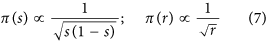When evaluated, equations (3)–(7) suggest that s > 0.79 and that the corresponding bound on |Q|, found by inverting the curve in Fig. 3, is |Q| < 0.59 p.p.b. without considering systematic uncertainties.

### Bounding the electric dipole moment

If we take Q = 0, but change the equation of motion, equation (2), to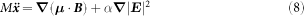to include the effects of electric polarizability, then we can reinterpret our experiment as setting a bound on the polarizability α of the anithydrogen atoms (in the absence of appreciable permanent monopole or dipole moments). This sets a limit of α < 3 × 106α0, at 68.3% confidence, not including systematic effects, where α0 = 4πε0 × 0.667 × 10−24cm3 is the known polarizability of hydrogen8. Note that effects due to polarizability will ultimately limit the precision of the stochastic methodology.

If instead we take Q = 0 and α = 0, and change the equation of motion to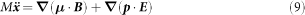then we can interpret our experiment as setting a bound on a permanent electric dipole moment p of antihydrogen. This gives a bound of 10−9e cm at 68.3% confidence not including systematic effects. If this moment were to come from, for instance, a positron dipole moment, then quantum corrections35 would loosen the bound to about 10−5e cm. This bound is many orders of magnitude greater than the current bound on the electron dipole moment of 9.7 × 10−29e cm (ref. 36).

### Identification of antihydrogen

Antihydrogen detection, and cosmic ray rejection, is based on previous work within the ALPHA experiment (see ref. 37). By taking the energy deposited in each strip of the SVD, clusters of activated strips can be summed into ‘raw hits’; raw hits from either side of a silicon module can be paired to make ‘hits’; hits can be compared in 3D space to create ‘tracks’; and tracks can be combined to point to an annihilation vertex.

The detector only detects the pions that are produced by the antiproton annihilations when antihydrogen atoms annihilate on the trap wall or on the very-low-density background gas; the detector is insensitive to the γ-rays produced by the simultaneous positron annihilations. We have developed many techniques for first eliminating antiprotons from our trap before the detection phase, and then discriminating between antihydrogen annihilations and the unlikely antiproton annihilations that might occur if some unbound antiprotons were still present1,17,25. We are confident that the events reported here are due to antihydrogen annihilations only.

To determine the best significance of antihydrogen detection against background, we fed multiple selection variables that measure the event shape and orientation into a MVA22,23,24. Complete reconstruction of the annihilation vertex is only part of the input to this MVA, because this method combines low- and high-level reconstruction data in a machine-learning algorithm. We used a large annihilation data sample and a large, independent, background data sample to train the machine-learning algorithm. The annihilation samples have fewer than 1% cosmic events and the background sample consists entirely of background events because the data are collected in the absence of antiprotons in the experiment. We then tested the performance of the MVA technique on a dedicated, separate test sample of data. Once trained, we used the MVA to analyse events that were detected during the experimental trials, and classified each event as either an antihydrogen annihilation event or as a background cosmic event. Note that we can tune the MVA differently for significance optimization during the stochastic/hold phase (2) and the release phase (3); for instance, we can decrease the false-negative rate where possible (increase the detection efficiency), at the cost of increasing the false-positive rate.

### MVA of the release phase (3)

With the MVA optimized for the 30-ms observation window of the release phase (3), (82.3 ± 0.2)% of the annihilation events are accepted with a background rate of 242 mHz ± 2%, which gives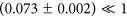expected cosmic rays in the observation window of both the null and stochastic trials. The results of these trials are reported in Table 1, and are the primary data used here.

### MVA of the stochastic/hold phase (2)

For the 10 null and 10 stochastic trials, events detected during the stochastic/hold phase (2) (before release) were fed into a differently trained MVA classifier that has a detector acceptance of (46.1 ± 0.3)% and a cosmic background rate of 6 mHz with 11% uncertainty (see Extended Data Table 1). Stochastic/hold phase events might have arisen from the escape of quasi-bound antiatoms, from annihilations on residual gas, or from ejection of the antiatoms by the stochastic acceleration. We performed a detailed statistical analysis to determine whether anything other than background needs to be introduced to account for the observed counts in Extended Data Table 1. That analysis (see Supplementary Information) shows that there is no reason to reject the assumption that these phase (2) counts are due to background, and thus do not provide reliable additional information regarding Q.

### Detector performance

Because consistency between the detector performance during the null and stochastic trials is critical for this analysis, we rigorously scrutinized and cross-checked the performance of the SVD with scintillation detectors placed on the outside of the apparatus. We examined several quality-of-data (QOD) criteria using both cosmic data from dedicated cosmic observation windows, and annihilation data from the mixing phase of experimental trials. We found no anomalous differences between the stochastic and null trials. The results of these tests27 are summarized in Extended Data Fig. 1.